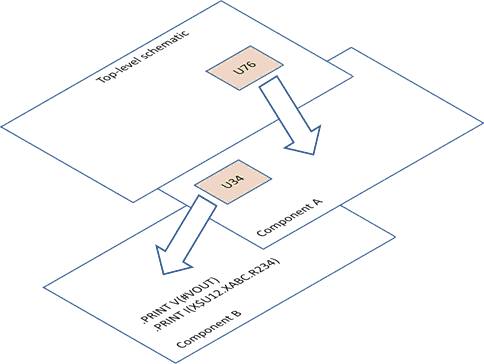# .PRINT

The .PRINT statement is used to specify the output variables to be recorded for printing/plotting. Note that with the SIMetrix/SIMPLIS, .PRINT specifies only data that is to be saved in the binary file and does not create an ASCII tabular output. .PRINT instructs SIMPLIS to send the specified data to the SIMetrix front end. SIMetrix saves the data as a vector in its binary file. The actual vector name is described in the following paragraphs.

The format of .PRINT is

`PRINT  var1 var2 ...`

where var1, var2, and ... are legal print variables. Forms of legal print variables and their meanings are listed below:

 Print Variable Definition SIMetrix Vector Name V(DName) Branch voltage across a two-terminal device in the current circuit. DName is the name of a device whose element keyword is one of the following:R, L, C, V, I, E, G, H, F, Q, S, !R, !L, and !C DName V(#NodeName) Voltage on mapped node NodeName. Mapped nodes are created using the .NODE_MAP statement. #NodeName I(DName) Branch current through a two-terminal device in the current circuit. DName is the name of a device whose element keyword is one of the following:R, L, C, V, I, E, G, H, F, Q, S, !R, !L, and !C DName#pinnamewhere pinname will be p for the first pin and n for the second pin I(DName#pinname) Current through a device pin. DName is the device name, and pinname is either a pin number or mapped pin name. If Dname is a subcircuit device and the subcircuit definition includes .NODE_MAP statements to map its external nodes to names, then those mapped names may be used for pinname. For example:```X1 1 2 3 SUB1 .SUBCKT SUB1 100 200 300 .NODE_MAP INP 100 .NODE_MAP INN 200 .NODE_MAP OUT 300 ... ... .ENDS SUB1 .PRINT I(X1#INP)``` The above .PRINT will instruct SIMPLIS to output the current into the pin connected to node 1 of X1. X1#INP V(Node1,Node2) Differential voltage from Node1 to Node2 where Node1 and Node2 are node names in the current circuit. V(Node1) Voltage from Node1 to node 0, the ground node. This form is allowable only in the main circuit and only if node 0 is present in the main circuit. Node1 V(Xname1.Xname2.DName) Branch voltage across the device named DName in the subcircuit referred to as Xname2 in the subcircuit referred to as Xname1 in the current circuit. The allowable device is same as those listed for the form of V(DName). Xname1.Xname2.DName I(Xname1.Xname2.DName) Branch current through the device named DName in the subcircuit referred to as Xname2 in the subcircuit referred to as Xname1 in the current circuit. Xname1.Xname2.DName#pinnamewhere pinname will be p for the first pin and n for the second pin. V(Xname1.Xname2.Node1,Node2) Differential voltage from Node1 to Node2 where Node1 and Node2 are node numbers in the subcircuit Xname2, in the subcircuit Xname1, in the current circuit. NODE_V Print all node voltages in the main circuit with respect to the ground node, node 0, in the main circuit. ALL Print all node voltages in the main circuit with respect to the ground node, node 0, in the main circuit and print all branch currents of the two-terminal devices in the main circuit. The two-terminal devices refer to those whose branch current can be printed through the form of I(DName)

In the normal case, no more than 200 output variables can be created for printing/plotting in one simulation. If more than 200 output variables are needed, the output variables can be generated through more than one pass of the simulation.

If the print variable NODE_V or ALL is used, then node 0 must be present in the main circuit. Although the number of output variables that are associated with NODE_V or ALL is obviously large, NODE_V or ALL are counted as one print variable in counting towards the maximum number of 200 print variables allowed in one simulation. Simulation can be substantially slower with NODE_V or ALL as a printing variable. In addition, if ALL is used as the print variable, other print variables defined in the main circuit or in any of its descendant subcircuits are ignored.

Two subtle points need to be understood when figuring out the SIMetrix vector names.  If DName or Xname as appeared in the .PRINT statement is a device/subcircuit name containing a dollar sign (\$), then all characters ahead of the first dollar sign and the first dollar sign are stripped in the corresponding DName or Xname in the SIMetrix vector name.  For example, the following .PRINT statement will result in a SIMetrix vector name of U12.XABC.R234#p

` .PRINT I(X\$U12.XABC.R234) `
In addition, the SIMetrix vector names shown for each type of print variables above assume that the .PRINT statement is defined in the top-level circuit/schematic.  If the .PRINT statement is defined in a subcircuit/component schematic, then the actual SIMetrix vector name will be prepended by a path prefix.  For example, look at the following two .PRINT statements:
```.PRINT V(#VOUT)
.PRINT I(X\$U12.XABC.R234)```

If these .PRINT statements are defined in the top-level circuit/schematic, the SIMetrix vector names would be #VOUT, and U12.XABC.R234#p, respectively.

In the diagram below, make these assumptions:

• The subcircuit/component schematic containing these two .PRINT statements is two-level down from the top-level circuit/schematic.
• The subcircuit/component schematic containing these two .PRINT statements can be reached from the top-level schematic by first descending into a component schematic named "Component A" with a reference designator of U76 and then descending into a component schematic named "Component B" with a reference designator of U34.The SIMetrix vector names associated with the same two .PRINT statements in this example would then be U76.U34.#VOUT and U76.U34.U12.XABC.R234#p, respectively.